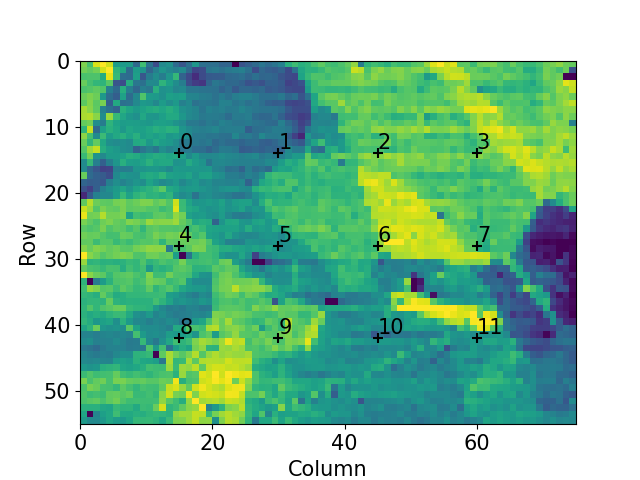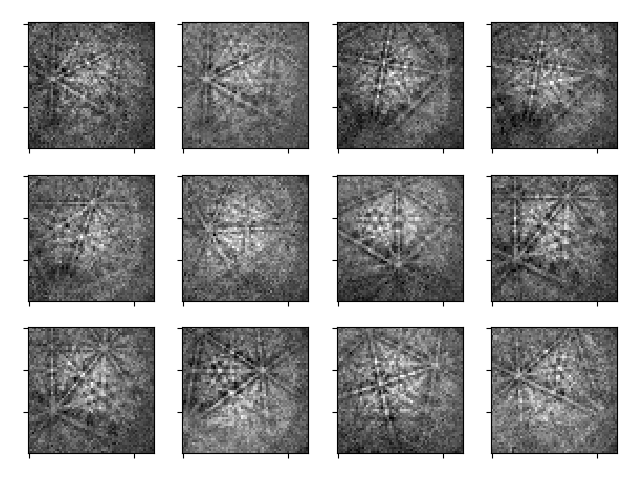# Extract patterns from a grid#

This example shows how to extract `EBSD` patterns from positions in a grid evenly spaced in navigation space.

••```<LazyEBSD, title: patterns Scan 1, dimensions: (75, 55|60, 60)>
<LazyEBSD, title: patterns Scan 1, dimensions: (4, 3|60, 60)>
```

```import hyperspy.api as hs
import kikuchipy as kp
import matplotlib.pyplot as plt

plt.rcParams["font.size"] = 15

# Silence progressbars
hs.preferences.General.show_progressbar = False

# Import data (lazily)
s = kp.data.nickel_ebsd_large(lazy=True)
print(s)

# Extract data, also getting the grid positions
s2, idx = s.extract_grid((4, 3), return_indices=True)
print(s2)

# Get virtual backscatter electron (VBSE) image from the intensities from the
# center of the detector, also slightly stretching the contrast
roi = hs.roi.RectangularROI(left=20, top=20, right=40, bottom=40)
vbse_img = s.get_virtual_bse_intensity(roi)
vbse_img.compute()  # Drop if data was not loaded lazily
vbse_img.rescale_intensity(dtype_out="float32", percentiles=(0.5, 99.5))

# Plot grid of extracted patterns
kp.draw.plot_pattern_positions_in_map(
idx.reshape(2, -1).T,
roi_image=vbse_img.data,
)

# Plot extracted patterns
s2.remove_static_background()
_ = hs.plot.plot_images(
s2, per_row=4, axes_decor=None, label=None, colorbar=None, tight_layout=True
)
```

Total running time of the script: ( 0 minutes 1.652 seconds)

Estimated memory usage: 9 MB

Gallery generated by Sphinx-Gallery